# Advantage of frequency domain analysis?

learner07
hello,

i am new here and this is my first post so please bear with me for any mistakes or so..

now my question is about time domain analysis and frequency domain analysis .

why should one convert from time domain into frequency domain by using fourier transform ? and what are the advantages of frequency domain analysis?

## Answers and Replies

Gold Member
Is this homework?

learner07
no i m interested to know why do u we actually deal with frequency domain a lot.....rather time domain which furnishes more information ?

learner07
please help me out i am new to this subject and want to learn it clearly..?

viscousflow
Frequency domain allows for techniques which could be used to determine the stability of the system. Also, these techniques can be use in conjunction with the S-domain (Laplace transform) which gives more insight to the stability of the system, transient response, and steady state response.

Gold Member
....time domain which furnishes more information ?

I wouldn't say this is the case.

Bob S
The time domain response of some circuits cannot be represented by Laplace functions. One example is the skin effect losses in coax cables, because the loss is proportional to √f.

Can you write the Fourier transform of a square wave? Can you write the Fourier transform of a square wave and include skin effect losses in a coax cable? See my post #11 in

Bob S

gnurf
why should one convert from time domain into frequency domain by using fourier transform ?
You should probably re-read the introduction chapter in your textbook, but maybe this example will help you on your way.

Look at this signal, y(t):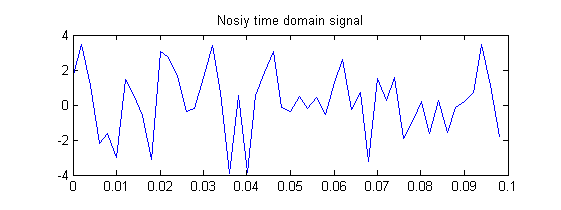Looks pretty random, right? For example, what frequency is that signal? The truth is that, unless you have a pure sinusoidal (e.g. a tuning fork), a real signal is often made up of many frequencies. The fourier transform is a tool that tells you which frequencies a signal consists of and to what degree (read: amplitude).

The noisy signal shown above is actually the sum of a sine wave, g(t) = sin(2πft) , and a normally distributed (a.k.a "white") noise function, n(t), with amplitude 1.7:

y(t) = g(t) + 1.7*n(t)

where the "white"-ness of n(t) means that its power is uniformly distributed across all frequencies (much like white light which consists of all colors). The frequency of g(t) is f = 100Hz. Because I've chosen the noise amplitude so that it doesn't drown out the 100Hz signal, a fourier transform of y(t) reveals the following picture: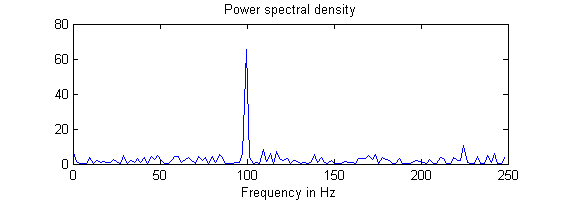The signal is decomposed into it's various frequency components. Pretty cool, I'd say. If you have matlab available, you can copypaste this code into a m-file and hit run. Play around with the noise amplitude and see how it affects the spectral density plot.

Code:
%------------------------------------------
% Power spectral estimation of noisy signal
%------------------------------------------

t = 0.0:0.002:0.5;

% Sine frequency
f1 = 100;

% Noise amplitude
a = 1.7;

% Generate the sine portion of signal
g = sin(2*pi*f1*t);

% Generate a normally distributed white noise
n = a*randn(size(t));

% Add the noise to the signal to get a noisy signal
y = g + n;

% Plot the noisy signal
subplot(211), plot(t(1:50),y(1:50)),
title('Nosiy time domain signal')

% Power spectral estimation:
yfft = fft(y,256)
len = length(yfft);
pyy = yfft.*conj(yfft)/len;
f = (500./256)*(0:127);
subplot(212), plot(f,pyy(1:128)),
title('Power spectral density'),
xlabel('Frequency in Hz')
EDIT: A perhaps more practical example would be a noisy signal where the noise was of a fixed frequency (60 Hz, for example). You could then use FFT to find the culprit and then add a notch filter to remove that noise.

Last edited:
2milehi
Here is a real life example of why to choose frequency domain over time domain.

On my Subaru late last winter, I added a turbo/intercooler and a MegaSquirt engine controller. The car ran good after some tuning but on a hot day in summer I heard the engine detonate badly. I connected my laptop's microphone to the knock sensor and recorded engine knock.

Here is the time domain signal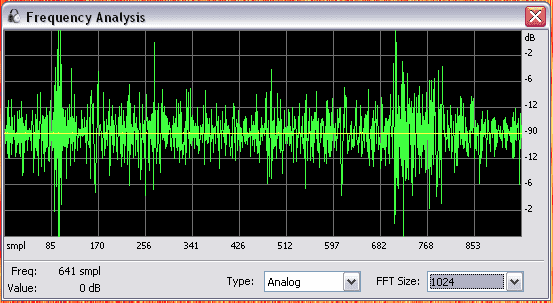Here is the frequency domain of that signal - ignore the yellow signal.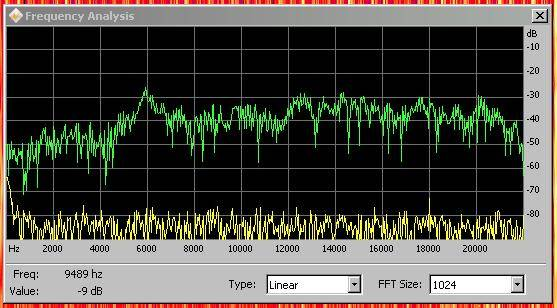And here is the frequency domain of regular engine noise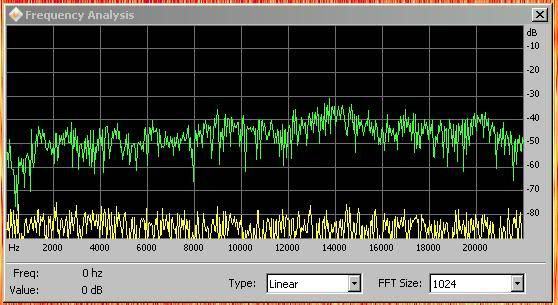It was very obvious for me to go after the spike at 5.9 kHz to determine if the engine is experiencing detonation.

There is also a rolling display of frequency domain.

Here is the engine being reved up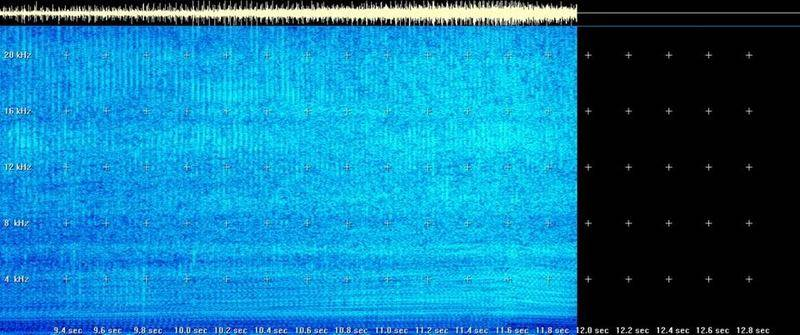and here is detonation while being reved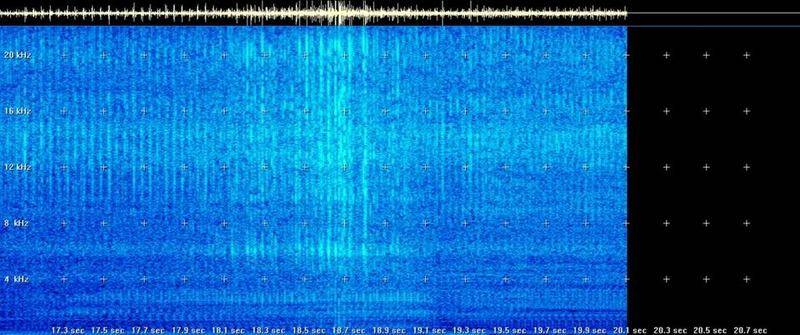The cool thing about frequency domain is that you get to see all the sounds.

Last edited:
Gold Member
I think this is largely practical and historical. Either domain is sufficient to describe any signal or channel characteristic, completely, but one is often more convenient than the other. 'The Maths' was known long before the engineering realisations came along.

In the beginning, there were oscillators and there were filters made of Ls, Rs and Cs. There were also moving coil meters. The natural way to analyse circuits was in the frequency domain. The performance of audio equipment was described in terms of 'frequency response' and 'harmonic distortion' - very satisfactorily, because phase/ timing is of secondary interest, subjectively, at audio frequencies.
TV came along and the effect of a channel on pulses became very relevant. The CRO gave a time domain display of a signal but, until the '90s, temporal filtering was not convenient most filtering was with lumped components. Simple delay line filters did exist but, until DSP arrived,you couldn't make transversal filters with arbitrary characteristics anything like as easily as you could make in 'the conventional' way.

The choice of f or t domain for any particular occasion depends upon what you need to find out and what equipment you have. You need to be able to slip out of one into the other, at will, to get the best understanding of the performance or design of any system.

jsgruszynski
The simple answer: the math and intuitions about how a circuit works are often simpler in the frequency domain rather than the time domain.